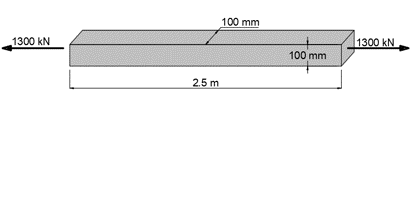# A steel bar pf length 2.5 m with a square cross section 100 mm on each side, is subjected to an...

## Question:

A steel bar of length 2.5 m with a square cross section 100 mm on each side, is subjected to an axial tensile force of 1300 kN. Assuming that E=200 GPa and {eq}\nu {/eq}=0.3, find:

a) The elongation of the bar,

b) The change in cross-sectional dimensions,

c) The change in volume.## Hooke's Law:

Hooke's Law states that under the elastic limit, the strain experienced by an object is proportional to the stress, as represented by Equation 1:

{eq}\epsilon=k\sigma \;(Equation\,1) {/eq}

where:

• {eq}\epsilon {/eq} = strain
• {eq}k {/eq} = proportionality constant
• {eq}\sigma {/eq} = stress

Some materials can withstand more stress than the others. Thus, the constant of proportionality depends on the level of elasticity of the material called the Young's Modulus of Elasticity. Equation 1 is then modified to:

{eq}\epsilon=\frac{\sigma}{E} {/eq}

## Answer and Explanation: 1

Become a Study.com member to unlock this answer! Create your account

Given:

• Length of the steel bar, {eq}(L)=2.5\,m=2500\,mm {/eq}
• Side of the square cross section, {eq}(w)=100\,mm {/eq}
• Tensile force,...

See full answer below.

#### Learn more about this topic:Young's Modulus: Definition & Equations

from

Chapter 8 / Lesson 3
17K

Young's modulus is a material property that tells you how stiff or stretchy a material is. In this lesson, learn how to calculate Young's modulus and what it can tell you about the material.